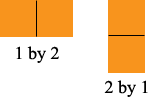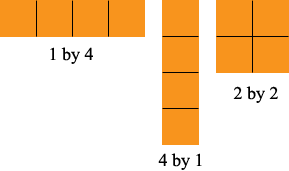SEARCH HOMEMath Central Quandaries & QueriesQuestion from Kim, a parent: My 4th grader has started working on rectangular array that shows 2 factors of a number. The problem reads on centimeter grid paper draw as many arrays as you can for each of the #'s 2,3,4, 5,6,7,,11,12,15 and 16. That would be great except I have no idea what they are talking about and I cannot offer any help to my daughter.Hi Kim,

For 2 I think the answer expected is the two arrays below.The number 2 has only two factors, 1 and 2. Notice that in describing them I wrote the number of rows first followed by the number of columns. This is the convention that mathematicians use.

For 4 there are 3 arrays.The prime numbers in your list, 2, 3, 5, 7 and 11 have only two arrays each but the rest have more than two arrays.

I hope this helps,
PennyMath Central is supported by the University of Regina and The Pacific Institute for the Mathematical Sciences.